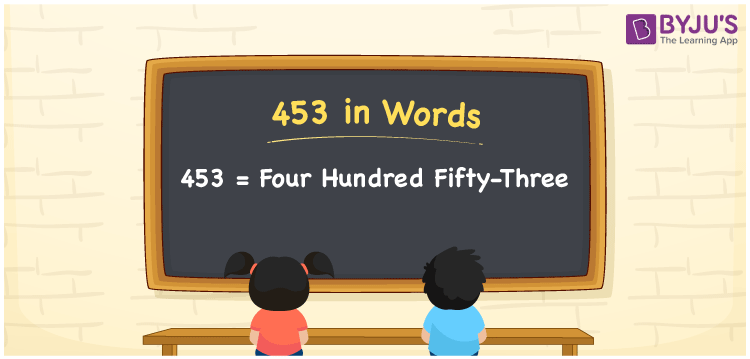# 453 in Words

453 in words is written as Four hundred fifty-three. In both the International System of Numerals and the Indian System of Numerals, 453 is written as Four hundred fifty-three. The number 453 is a Cardinal Number as it represents some quantity. For example, the “cost of the t-shirt is rupees 453”.

 453 in Words Four hundred fifty-three Four hundred fifty-three in Number 453

## 453 in English Words

We write 453 in English Words using the letters of the English alphabet. Therefore, we read 453 in English as “Four hundred fifty-three”.## How to Write 453 in Words?

To write 453 in words, we shall use the place value chart. In the place value chart, write 4 in the hundreds, 5 in the tens, and 3 in the ones, respectively. Now let us make a place value chart to write the number 453 in words.

 Hundreds Tens Ones 4 5 3

Thus, we can write the expanded form as

4 × Hundred + 5 × Ten + 3 × One

= 4 × 100 + 5 × 10 + 3 × 1

= 400 + 50 + 3

= 453

= Four hundred fifty-three.

453 is a natural number, the successor of 452 and the predecessor of 454.

453 in words – Four hundred fifty-three

• Is 453 an odd number? – Yes
• Is 453 an even number? – No
• Is 453 a perfect square number? – No
• Is 453 a perfect cube number? – No
• Is 453 a prime number? – No
• Is 453 a composite number? – Yes

## Frequently Asked Questions on 453 in Words

Q1

### How to write 453 in words?

453 in words is written as Four hundred fifty-three.
Q2

### How to write 453 in the International and Indian System of Numerals?

In both, the system of numerals, 453 in words, is written as Four hundred fifty-three.
Q3

### What is the successor of 453?

The successor of 453 is 454.